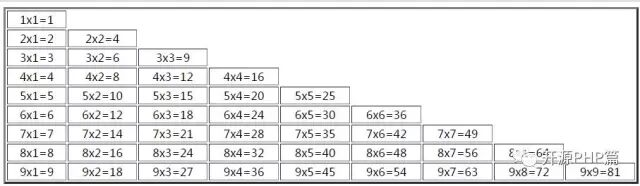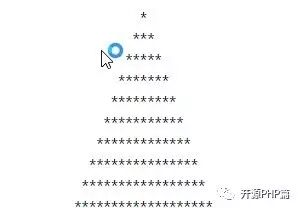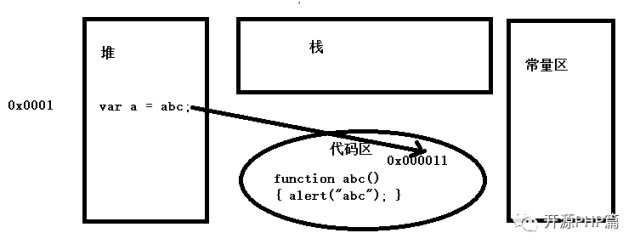# 深入浅出学习javascript(一)

1、变量

var系统内部的关键字，用来定义变量名，代表数据类型的名字。

只能包含数字，字母，下划线
变量名不能以数字开头
系统内部的关键字
大小写区分，如果命名的时候是小写，那么在用的时候必须也是小写
建议命名变量名的时候要让这个变量名有意义

<script>
var a = 10;
var str = "我是中国人";
var b = 0.9;
</script>

2、与输出相关的小知识点

document.write(变量名)：输出内容放在页面上，可以通过浏览器调式看到。
typeof(变量名)：得到数据的类型。

console.log(object[, object, ...])：在控制台输出一条消息。如果有多个参数，输出时会用空格隔开这些参数。

console.debug(object[, object, ...])：在控制台输出一条消息，包含一个指向代码调用位置的超链接。假如是直接在控制台输入该命令，就不会出现超链接（和console.log()一样）。
console.info(object[, object, ...])：在控制台输出一条带有“信息”图标的消息和一个指向代码调用位置的超链接。
console.warn(object[, object, ...])：在控制台输出一条带有“警告”图标的消息和一个指向代码调用位置的超链接。
console.error(object[, object, ...])：在控制台输出一条带有“错误”图标的消息和一个指向代码调用位置的超链接。
console.assert(expression[, object, ...])：测试表达式expression是否为真。如果不是真，会在控制台写一条消息并抛出异常
console.dir(object)：以列表形式输出一个对象的所有属性，有点和你查看DOM窗口相类似。
console.dirxml(node)：输出一个HTML或者XML元素的XML源代码。和你在HTML窗口看到的相似。

console.trace()：在调用的点处打印JavaScript执行的交互式堆栈跟踪。堆栈跟踪详细说明了堆栈上的函数，以及作为传递的值每个函数的参数。 您可以单击每个函数将其带到Scripttab中的源， 并单击每个参数值以在DOM或HTML选项卡中检查它。
console.group(object[, object, ...])：输出一条消息，并打开一个嵌套块，块中的内容都会缩进。调用console.groupEnd()关闭块。该命令可以嵌套使用。
console.groupEnd()：关闭最近一个由console.group打开的块。
console.time(name)：创建一个名字为name的计时器，调用cosole.timeEnd(name)停止计时器并输出所耗时间（毫秒）。
console.timeEnd(name）

3.数据类型

4、js的运算符

i++，++i：（都是表示变量i自加1）
i--，--i：（都是表示变量i自减1）

5、js的注释（和C/C++、Java等语言一致）

//单行注释
/**/多行注释

6、一个小案例

<script>
document.write("<h1>I</h1>");
document.write("<h2>Love</h2>");
document.write("<h3>You</h3>");
</script>

7、js中传值的方式

1、js的流程控制
顺序结构

代码都是从上往下一步一步执行的

分支结构

if结构
if…else…结构
if…else if…else…结构

switch结构

循环结构

for循环结构
while循环结构
do…while…循环结构

2、js的流程控制与循环结构小案例<script>
document.write("<table border='3' width='900' align='center'>");
for(var i = 1; i <= 9; i++)
{
document.write("<tr>");
for(j = 1; j <= i; j++)
{
document.write("<td align='center'>");
document.write(i +"x" + j + "=" + i*j);
document.write("</td>");
}
document.write("</tr>");
}
document.write("</table>");
</script><script>
document.write("<p align='center'>");
for(var i = 1; i < 100; i++)
{
for(var j = 1; j <= (2 * i -1);  j++)
{
document.write("*");
}
document.write("<br/>");
}
document.write("</p>");
</script>

1、什么是函数

2、定义函数语法：
function 函数名([参数1,参数2...])

{
函数执行部分;
return 表达式;
}

3、调用函数语法：
函数名(实际参数1，实际参数2 ...);

4、函数名：
函数名不可以是系统内部关键字
要让函数名有意义
5、形式参数：
形式参数只能名字的形式，直接写出名字，这个名字可以认为是变量名（不用加var）在函数内部直接用在形式参数写好的名字即可，这个名字代表的数据，是调用函数时候的实际参数
6、实际参数：
只能是一个具体的数据（或者用变量名代替），实际参数的数据的名字就是定义函数的名字
7、函数体：
写的程序是有目的性的，写这个函数要完成什么功能而写
8、函数的执行：
定义好函数之后是不会去执行的，在代码运行到调用函数的时候才会去定义函数的位置执行对应的代码。

9、函数的作用域：
局部变量：在函数内部用var定义的变量，只能在函数内部使用
全局变量：在函数外部用var定义的变量，在任何地方都可以使用

10、函数的递归（递归函数）
Function abc(){abc();}
abc();

11、关于函数的小案列

<script>
function pyramid(n)
{
document.write("<p align='center'>");
for(var i = 1; i < n; i++)
{
for(var j = 1; j <= (2 * i -1);  j++)
{
document.write("*");
}
document.write("<br/>");
}
document.write("</p>");
}
</script>
<script>
pyramid(10);
</script>

<script>
function digui(n)
{
if(n < 1)
{
return ;
}
else
{
digui(n - 1);
document.write(n + "<br/>");
}
}
digui(100);
</script>

12、匿名函数

<script>
(function ()
{

})();
</script>

13、函数在内存中的存储形式<script>
function abc()
{
}

var a = abc;
a();
</script>

<script>
function abc()
{
}
abc();
</script>

<script>
var a = function abc()
{
}
a();
</script>

14、系统内部的一些函数

parseInt（参数），通过函数parseInt获得参数的整数位
parseInt（9,.2）=>9
parseInt(9.9) ;=>9
parseInt(9.0) ;=>9
parseInt(“9.3”);
parseInt(“9.3abc”)
parseInt(“abc9.3”);
parseFloat(参数);通过函数parseFloat获得参数的浮点数
parseFloat(9.2);=>9.2
parseFloat(9.922);=>9.922(0.922)
parseFLoat(“9.23abc”);
parseFloat(“9.23”);
parseFloat(“abc9.23”);
isNaN：是一个不是数字的数据，布尔值
var m=isNaN(23);
var m=isNaN(“23”);
var m=isNaN(“abc”);
NaN:不是一个数字

1、什么是数组

2、数组的定义

var 数组名称 = [数组元素1，数组元素2，数组元素3…];

var 数组名称 = new Array(数组元素1，数组元素2，数组元素3…);

var 数组名称 = new Array(3); 这个定义方式不推荐使用
数组名称 = 数组元素1;
数组名称 = 数组元素2;
数组名称 = 数组元素3;

var arr1 = ['紫霞仙子', '东方不败', '杨贵妃'];

var  arr2 = new Array('西施', '貂蝉', '王昭君');

var arr3 = [['成都', '汉中', '绵阳'],['贵阳','遵义','六盘水'],['哈尔滨','大庆','齐齐哈尔']];

3、数组的访问

4、获取数组的长度并遍历数组元素

<script>

var arr = ['大锤', '小s', '小美'];

for(var i = 0; i < arr.length(); i++)

{

document.write(arr[i] + "</br/>");

}

</script>

for(var i in 数组) {}

<script>

var arr = ['大锤', '小s', '小美'];

for(var i in arr)

{

document.write(arr[i] + "</br/>");

}

</script>

5、数组排序

6、数组相关的几个案例

<script>
var arr = [[20, 15, 25],[10, 50, 35],[25, 65, 45]];
var num = 0
for(var i = 0; i < arr.length; i++)
{
for(var j = 0; j < arr[i].length; j++)
{
num = num + arr[i][j];
}
}
document.write(num);
</script>

<script>
var arr = [[20, 15, 25],[10, 50, 35],[25, 65, 45]];
var maxvlaue = arr;
var index1 = 0;
var index2 = 0;
for(var i = 0; i < arr.length; i++)
{
for(var j = 0; j < arr[i].length; j++)
{
if(maxvlaue < arr[i][j])
{
maxvlaue = arr[i][j];
index1 = i;
index2 = j;
}
}
}
document.write("数组最大值为"+ maxvlaue + "," + "下标为" + index1 + "," + index2);
</script>

7、文本下标

<script>

var arr = ['a', 'b', 'c'];

arr['first'] = 'd';

arr['second'] = 'e';

document.write(arr['first']);

document.write(arr['second']);

</script>

<script>

var arr = ['a', 'b', 'c'];

arr['first'] = 'd';

arr['second'] = 'e';

</script>

8、与数组相关的函数

<script>
var province = ['请选择', '贵州省', '山东省', '黑龙江省'];
var city = [[], ['贵阳', '毕节', '安顺', '六盘水', '遵义'], ['济南', '青岛', '菏泽', '聊城', '烟台', '蓬莱'], ['哈尔滨', '大庆', '齐齐哈尔', '牡丹江' , '黑河']];
function createProvince()
{
for(var i in province)
{
var op = new Option(province[i], i);
}
}

{
createProvince();
}
</script>
<select id = "province"></select>
<select id = "city"></select>

<script>
function func()
{
var obj = document.getElementById("id1");
var imgs = obj.value.lastIndexOf(".");
var hz = obj.value.substr(imgs+1);
hz = hz.toLowerCase();
if(hz == "jpg" || hz == "png" || hz == "gif")
{
}
else
{
}
}
</script>
<input type="file" id="id1">
<input type="button" value="点击上传"  onclick="func()">

Math.pow(x,y);求的X的Y次方
Math.ceil(n);向上取整:不小于这个数的最小整数
Math.floor(n);向下取证:不大于这个数的最大整数
Math.round();四舍五入
Math.abs(n);取n的绝对值
Math.max(23,12);取最大值
Math.min(12,22);取最小值
Math.random();取得0到1之间的随即数，注意：能取到0但是不能取到1

<script>
function fun(num1,  num2,  n)
{
for(var i = 0; i < n; i++)
{
var m = Math.random();
m = m * (num2 + 1) + num1;
m = Math.floor(m);
document.write("m = " + m);
}
}
fun(10, 20, 6);
</script>

obj.toLocaleString();以当地时间格式显示
obj.getFullYear();得到的是年份
obj.getMonth();得到月份
obj.getDate();得到日期
obj.getHours();得到小时
obj.getMinutes();得到分钟数
obj.getSeconds();得到的是秒
obj.getMilliSeconds()得到毫秒
obj.getTime();以毫秒值的形式显示
obj.getDay();获得星期

obj.setFullYear();设置年份
obj.setMonth();设置月份
obj.?setDate();设置日期
obj.setHours();设置小时
obj.setMinutes();设置分钟数
obj.setSeconds()设置秒
obj.setMilliSeconds();设置毫秒

\             转移字符
‘              单引号
“              双引号
\r             换行
\n            回车
\t            tab符

1、鼠标事件：
Onclick：单击事件
Onmouseover：鼠标放上
Onmouseout：鼠标离开
OnmouseDown：鼠标按下

OnmouseUp：鼠标抬起
Ondblclick：双击
Onmousemove：鼠标移动

2、表单事件
Onsubmit：提交事件
Onfocus：获得焦点
Onblur：失去焦点
Onreset：重置事件

3、键盘事件：
OnkeyUp：键盘按键抬起
OnkeyDown：键盘按键按下
OnkeyPress：键盘按键按一次
</body>

4、Event对象：当事件发生的时候相关的信息
Event.clientX：鼠标移动时X坐标
Event.clientY：鼠标移动时Y坐标
Event.target：事件源

5、HTML和CSS属性的取值和赋值
(1)、html
取值：Var n=obj.html属性名
赋值：Obj.html属性名=m;
(2)、css
取值var n=obj.style.CSS属性名。例如：obj.style.margin; obj.style.borderBottom
赋值：obj.style.CSS属性名。例如：obj.style.margin="30px";0248